# Two Straight Flushes

Let's now look at the probability that 2 players end up with straight flushes. For simplicity let us use hearts as the suit but it is clear there is nothing special about hearts. If there are 3 hearts in the board and 2 players have straight flushes, the only possibility is that the ranks of the hearts on board have the form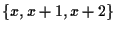, 1 player has x-2and x-1 of hearts, and the other player has x+3 and x+4 of hearts. Thus, the possible range for x is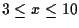. Let's count the number of boards which look like this. There are 4 choices for suit, 8 choices for the rank x andchoices for the other 2 cards comprising the board. The product of these 3 numbers is 23,712.

For each such board, there are 4 hearts which must go to 2 players in a fixed way in order for 2 straight flushes to arise. There are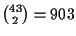choices for the completion of 1 set of hearts to a hand, andfor the completion of the other 2 hearts to a hand. This produces 740,460 ways for 2 players to have hands which give 2 players straight flushes for a fixed board of the type under discussion. For the other 8 players, we are choosing 32 cards from 39 and then partitioning them in (5) ways to give us semi-deals. Thus, the number of semi-deals with 2 players having straight flushes, when there are 3 suited cards on board, is the product of 23,712,, (5) and 740,460. We get

 16,010,830,956,990,783,872,058,420,189,870,000,000 (9)

semi-deals.

Now let us assume there are 4 hearts on board and 2 players have straight flushes. We have to determine the number of rank sets for 4 hearts which allow 2 players to have straight flushes. One possibility is that there are 4 successive ranks of the form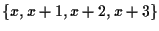, where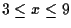. There are 7 sets of that form. Another possibility is 3 successive ranks together with an isolated rank, that is, a rank set of the form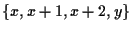, where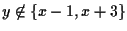. The range for x is from 3 through 10. When x=3 or x=10, there are 3 ranks which y cannot take on, but for any other value of x, there are only 2 ranks from which y is excluded. Thus, there are 62 rank sets with 3 successive ranks.

Now consider 2 successive ranks occurring but not 3 consecutive ranks. The only way 2 players can have straight flushes in this case is if the rank set has the form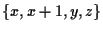, where y<x-1 and z>x+2. The possible range for x is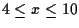. There are 2 possible values for y, namely, x-2 or x-3, and 2 possible values for z. Thus, there are 28 rank sets.

Finally, 4 hearts on board with no successive ranks do not allow 2 players to have straight flushes so this pattern cannot occur. Thus, we have 97 legal rank sets with 4 suited cards on board. There are 4 choices for the suit, 39 choices for the fifth card, 740,460 ways for 2 players to have hands which give 2 straight flushes, and the number of semi-deals in (5) for the remaining 8 players. We take the product of all these numbers to give us

 664,292,310,442,510,596,237,641,250,000 (10)

semi-deals for which 2 players have straight flushes when there are 4 suited cards on board.

We now move to the case there are 5 suited cards on board. The primary work is to determine how many rank sets there are which allow 2 players to have qualifying straight flushes. If there are 5 successive ranks, then 2 players can have qualifying straight flushes. In this case, the range for the smallest card is from 3 through 8. Hence, there are 6 rank sets being counted here. Now suppose there are 4 successive ranks x, x+1,x+2,x+3 and a fifth rank y. The possible range for x is. When x=3 or x=9, there are 3 values excluded for y. In the other cases, there are only 2 values excluded for y. So we have 47 rank sets which work when there are 4 successive ranks.

Now suppose the rank set has the form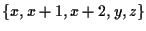. Clearly,must be the case as there is not room to fit 2 qualifying straight flushes otherwise. When x=3 or x=10, y,z may be any 2 ranks chosen from a 7-set so there are 21 choices. When x=4 or x=9, there are 27 choices because we cannot choose both A and 2 in the former case, or both K and A in the latter case. For all other values we can choose any 2 from an 8-set giving us 28 choices. Altogether we have 208 rank sets which work when there are 3 successive ranks.

Now we may assume the longest sequence of successive ranks is 2. First consider the situation in which there are 2 such sequences, that is, we have ranks x,x+1 and y,y+1. Without loss of generality we may assume x is the smallest of the 4 ranks. If the fifth rank z is smaller than x, then one qualifying player must form a straight flush using z,x,x+1but NOT hold x+2 in her hand as no other player would be able to have a straight flush. So the 2 possibilities are x-3,x,x+1 and x-2,x,x+1with the player holding x-2,x-1 and x-3,x-1, respectively. This then forces y,y+1 to be either x+3,x+4 or x+4,x+5, where the second player's cards are uniquely determined in both cases for her to have a qualifying straight flush. Since x-3 can be as small as ace and x+5can be as big as ace, the possible range for x is 4 through 9 and we get 24 rank sets. Next, if z lies between x+1 and y, then we can work out that the possibilities are x,x+1,x+3,x+5,x+6, x,x+1,x+3,x+6, x+7, x,x+1,x+4,x+6,x+7, and x,x+1,x+4,x+7,x+8. Again there are 24 possibilities. If z is larger than y+1, we get a reflection of the first case giving us another 24. So we have 72 rank sets with 2 sequences of successive ranks.

The next case is only 1 sequence of successive ranks x,x+1. The other 3 isolated ranks cannot all be smaller than x or all larger than x as it is easy to see this makes it impossible for 2 players to have straight flushes. Assume exactly 1 rank is smaller than x. It is easy to see it must be either x-3 or x-2. The larger rank is either x+3 and something of rank x+5 and bigger, or x+4 and something bigger than x+5. Actually listing them all leads to finding there are 68 like this. By symmetry there are another 68 when exactly 1 rank is larger than x+1. This gives us 136 rank sets in this case.

Finally, we move to rank sets with no successive ranks. There can be 2 players with straight flushes only when the rank set has the form x,x+2,x+4,x+6,x+8. The range for x is ace through 6 so there are 6 rank sets of this type. Adding all of the rank sets gives us 475 rank sets. Since there are 4 possible suits, the total number of rank sets allowing 2 qualifying straight flushes when there are 5 suited cards in the board is 1,900. As before, there are 740,460 ways of producing 2 hands which yield the 2 straight flushes for each fixed board. We then multiply by (5) to take into account the remaining 8 hands. This yields

 83,409,687,406,870,878,459,656,250,000 (11)

semi-deals for which 2 players have qualifying straight flushes when there are 5 suited cards on board.

Adding (9), (10) and (11) yields

 16,010,831,704,692,781,721,439,894,887,167,500,000 (12)

semi-deals for which 2 players have qualifying straight flushes. Dividing (12) by (3) yields a probability of .00002762184811 that 2 players have qualifying straight flushes. This is roughly 1 in 36,000.

Theorem 3.1   The probability of a semi-deal occurring in Omaha for which 2 players have straight flushes is .00002762184811.

Brian &
2000-11-02